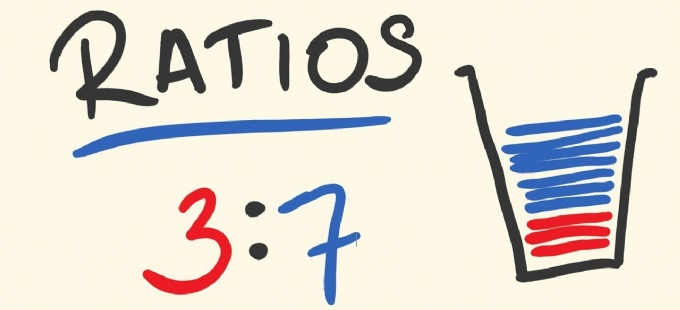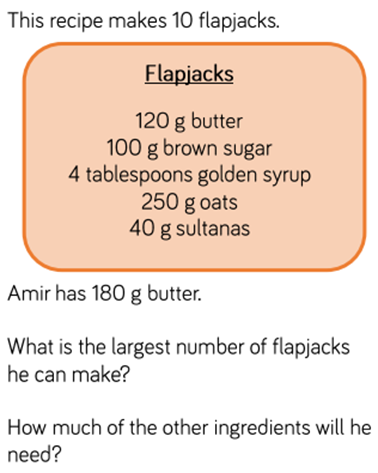# RatioOur Final Week of Ratios...

We have looking at Ratios and proportion for the last couple of weeks.  We have been looking at how to write ratios correctly using the ratio symbol :, we have been calculating ratios, using scale factors and solving ratio and proportion problems.

There has been some excellent problems solving using ratios from the Jaguars this week too.

Go ChallengeThe largest amount Amir can make 15 flapjacks. 60 divided by 12 = 5 + 10 = 15 flapjacks. Ingredients are:

180g butter

150g brown sugar

6 tablespoons of golden syrup

375g oats

60g sultanas